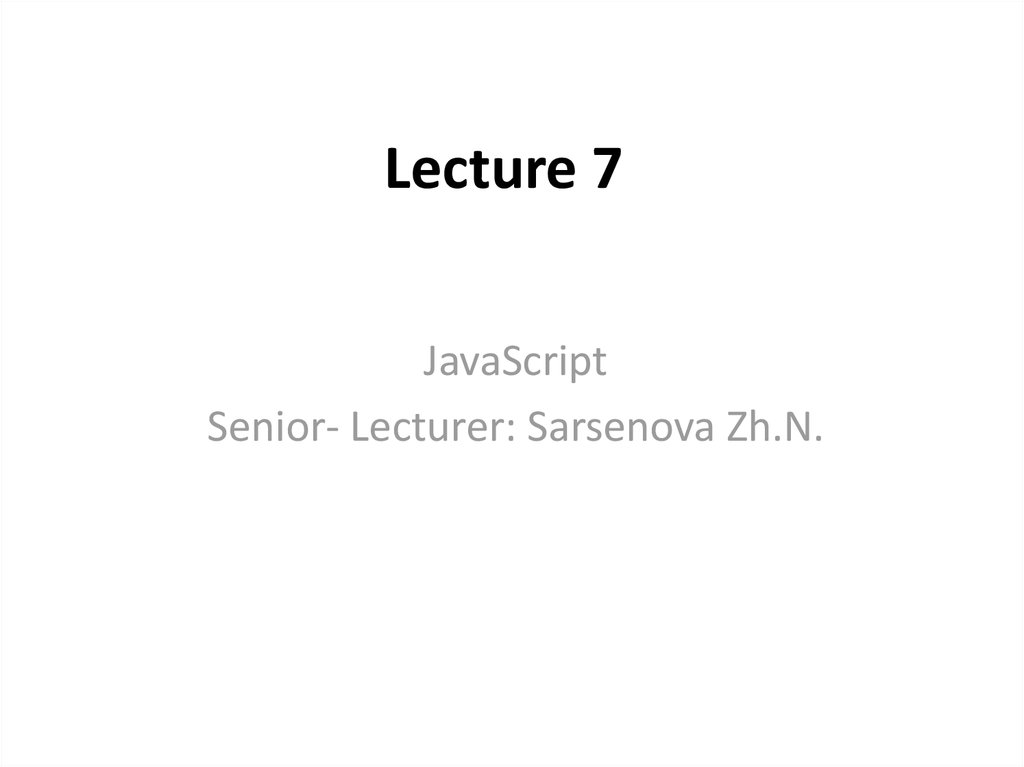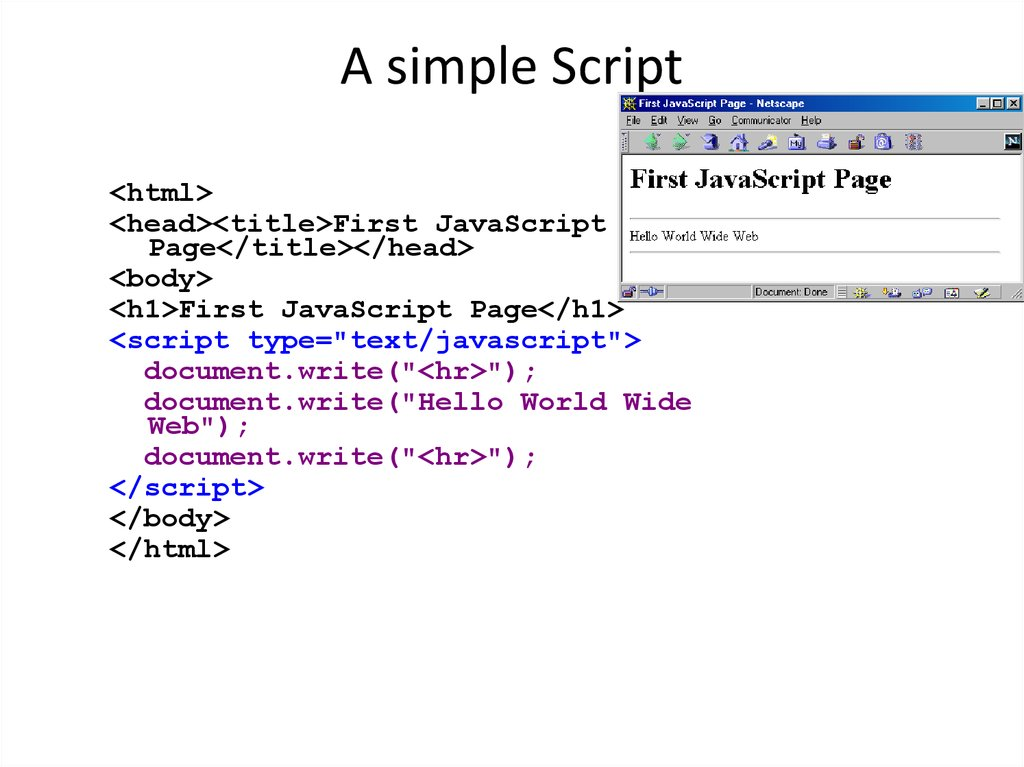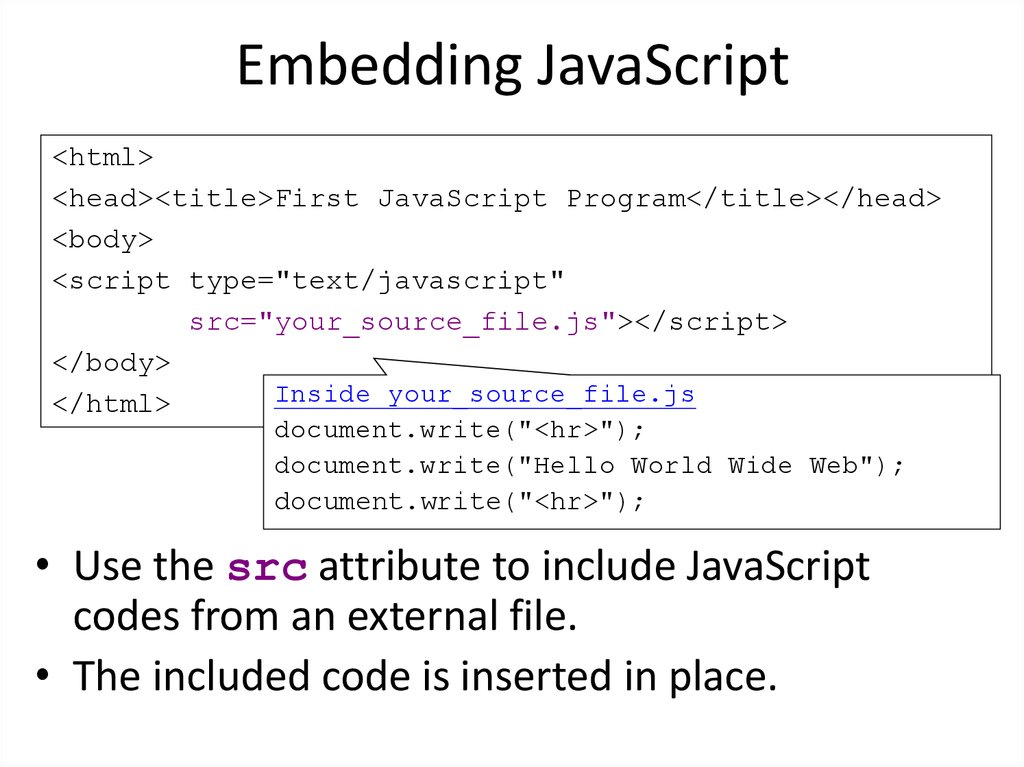# A simple Script

## 1. Lecture 7

JavaScript
Senior- Lecturer: Sarsenova Zh.N.

## 2. A simple Script

<html>
<body>
<h1>First JavaScript Page</h1>
<script type="text/javascript">
document.write("<hr>");
document.write("Hello World Wide
Web");
document.write("<hr>");
</script>
</body>
</html>

## 3. Embedding JavaScript

<html>
<body>
<script type="text/javascript"
src="your_source_file.js"></script>
</body>
Inside your_source_file.js
</html>
document.write("<hr>");
document.write("Hello World Wide Web");
document.write("<hr>");
• Use the src attribute to include JavaScript
codes from an external file.
• The included code is inserted in place.

• Confirm box
• Prompts box

## 5. alert(), confirm(), and prompt()

<script type="text/javascript">
confirm("Are you OK?");
prompt("How old are you?","20");
</script>

## 6. The typeof operator

• typeof operator used to find the type of a
JavaScript variable.

## 8. Undefined data type

• In JavaScript, a variable without a value, has the
value undefined. The typeof is also undefined.

## 9. Null

• In JavaScript null is "nothing". It is supposed to be
something that doesn't exist.
• Unfortunately, in JavaScript, the data type of null is
an object.

## 12. Line Breaks

• To display line breaks inside a popup
box, use a back –slash followed by the
character n.
• Example: alert(“Hello\n How are you?”);

## 13. JavaScript Objects

• Objects in real life is for instance
Students
• Properties: name, ID, weigh, height etc.
• Methods: eat, speak, walk, read, do
something and etc.

## 14. JavaScript Objects

• You have already learned that JavaScript
variables are containers for data values.

## 15. Objects

• Objects are variables too.
• Objects can contain many values

## 16. Example

This code assigns many
values (Fiat, 500, white)
to a variable named car
The values are written as
name: value pairs (name
and value separated by a
colon)

## 17. Object Properties

• The name: values pairs are called
properties.
Property
Property
value

## 18. Object Methods

• Methods are actions that can be
performed on objects.
• Methods are stored in properties as
function definitions

• 2 ways
Or

## 21. Accessing Object methods

• ObjectName.methodName()

## 23. Do Not Declare Strings, Numbers, and Booleans as Objects!

• When a JavaScript variable is declared
with the keyword "new", the variable is
created as an object:

## 24. Conditional Statements

• Very often when you write code, you
want to perform different actions for
different decisions. You can use
conditional statements in your code to
do this.

## 25. Types of conditional statements

• if statement - use this statement if you want to
execute some code only if a specified condition is
true
• if...else statement - use this statement if you want
to execute some code if the condition is true and
another code if the condition is false
• if...else if....else statement - use this statement if
you want to select one of many blocks of code to be
executed
• switch statement - use this statement if you want to
select one of many blocks of code to be executed

## 26. If statement Syntax and example

• If (expression) {
Statements to be executed if expression is
true
}

## 30. Switch Statement

• You should use the Switch statement if
you want to select one of many blocks of
code to be executed.

## 33. The break Keyword

• When JavaScript reaches
a break keyword, it breaks out of the
switch block.
• This will stop the execution of more code
and case testing inside the block.
• When a match is found, and the job is
done, it's time for a break. There is no
need for more testing

## 34. The default Keyword

• The default keyword specifies the code
to run if there is no case match:

## 35. JavaScript Math Objects

• allows you to perform mathematical tasks on numbers
• Math.round(x) returns the value of x rounded to its nearest integer
• Math.round(4.7); // returns 5
Math.round(4.4); // returns 4:
• Math.pow(x, y) returns the value of x to the power of y:
• Math.pow(8, 2); // returns 64
• Math.sqrt(x) returns the square root of x:
• Math.sqrt(64); // returns 8
• Math.abs(x) returns the absolute (positive) value of x:
• Math.abs(-4.7); // returns 4.7
• Math.ceil(x) returns the value of x rounded up to its nearest integer:
• Math.ceil(4.4); // returns 5
• Math.floor(x) returns the value of x rounded down to its nearest integer:
• Math.floor(4.7); // returns 4
• Math.min() and Math.max() can be used to find the lowest or highest value in a list
of arguments:
• Math.min(0, 150, 30, 20, -8, -200); // returns -200
• Math.max(0, 150, 30, 20, -8, -200); // returns 150
• Math.random() returns a random number between 0 (inclusive), and 1 (exclusive):
• Math.random();

## 36. Math Properties (Constants)

• Math.E
// returns Euler's number
Math.PI
// returns PI
Math.SQRT2 // returns the square root of 2
Math.SQRT1_2 // returns the square root of 1/2
Math.LN2 // returns the natural logarithm of 2
Math.LN10 // returns the natural logarithm of 10
Math.LOG2E // returns base 2 logarithm of E
Math.LOG10E // returns base 10 logarithm of E

## 37. JavaScript Random Integers

• Math.random() used with Math.floor() can be used
to return random integers.
• Math.floor(Math.random() * 10); //returns a
number between 0 and 9